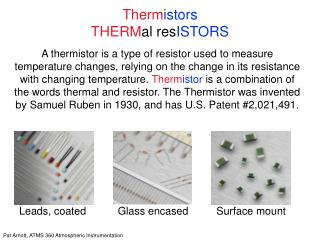DownloadDownload PresentationTherm istors THERM al res ISTORS

# Therm istors THERM al res ISTORS

Télécharger la présentation## Therm istors THERM al res ISTORS

- - - - - - - - - - - - - - - - - - - - - - - - - - - E N D - - - - - - - - - - - - - - - - - - - - - - - - - - -
##### Presentation Transcript

1. ThermistorsTHERMal resISTORS A thermistor is a type of resistor used to measure temperature changes, relying on the change in its resistance with changing temperature. Thermistor is a combination of the words thermal and resistor. The Thermistor was invented by Samuel Ruben in 1930, and has U.S. Patent #2,021,491. Leads, coated Glass encased Surface mount

2. ThermistorsTHERMal resISTORS Thermistors are made of semiconductor materials (metallic compounds including oxides such as manganese, copper, cobalt, and nickel, as well as single-crystal semiconductors silicon and germanium). Contrast <<--->> Common carbon resistors, made from carbon powder mixed with a phenolic binder glue. Leads, coated Glass encased Surface mount

3. ThermistorsTHERMal resISTORS Assume a simple linear relationship between resistance and temperature for the following discussion: ΔR = k ΔT where ΔR = change in resistance ΔT = change in temperature k = first-order temperature coefficient of resistance Source: http://en.wikipedia.org/wiki/Thermistor

4. ThermistorsTHERMal resISTORS Thermistors can be classified into two types depending on the sign of k. If k is positive, the resistance increases with increasing temperature, and the device is called a positive temperature coefficient (PTC) thermistor, Posistor. If k is negative, the resistance decreases with increasing temperature, and the device is called a negative temperature coefficient (NTC) thermistor. Source: http://en.wikipedia.org/wiki/Thermistor

5. ThermistorsTHERMal resISTORS Resistors that are not thermistors are designed to have the smallest possible k, so that their resistance remains almost constant over a wide temperature range. Source: http://en.wikipedia.org/wiki/Thermistor

6. ThermistorsTHERMal resISTORS Thermistor-choice is based on the nominal resistance you want at the operating temperature range, on the size, and on the time constant. Time constants are about 5 - 10 seconds. (Check this out with your thermistor). Source: http://www.science.uwaterloo.ca/~jddmarti/p352_w2007/Thermistor_50K.pdf

7. ThermistorsTHERMal resISTORS Source: http://www.science.uwaterloo.ca/~jddmarti/p352_w2007/Thermistor_50K.pdf

8. ThermistorsTHERMal resISTORS Example Applications: Temperature measurement. Time delay (self heating from large current ‘opens’ the thermistor so it can be used as a slow switch). Heating = i2 R where R is the resistance and i is the current. Surge suppression when a circuit is first energized. Current needs to flow through the thermistor for awhile to heat it so that it ‘opens’, and acts again as a switch.